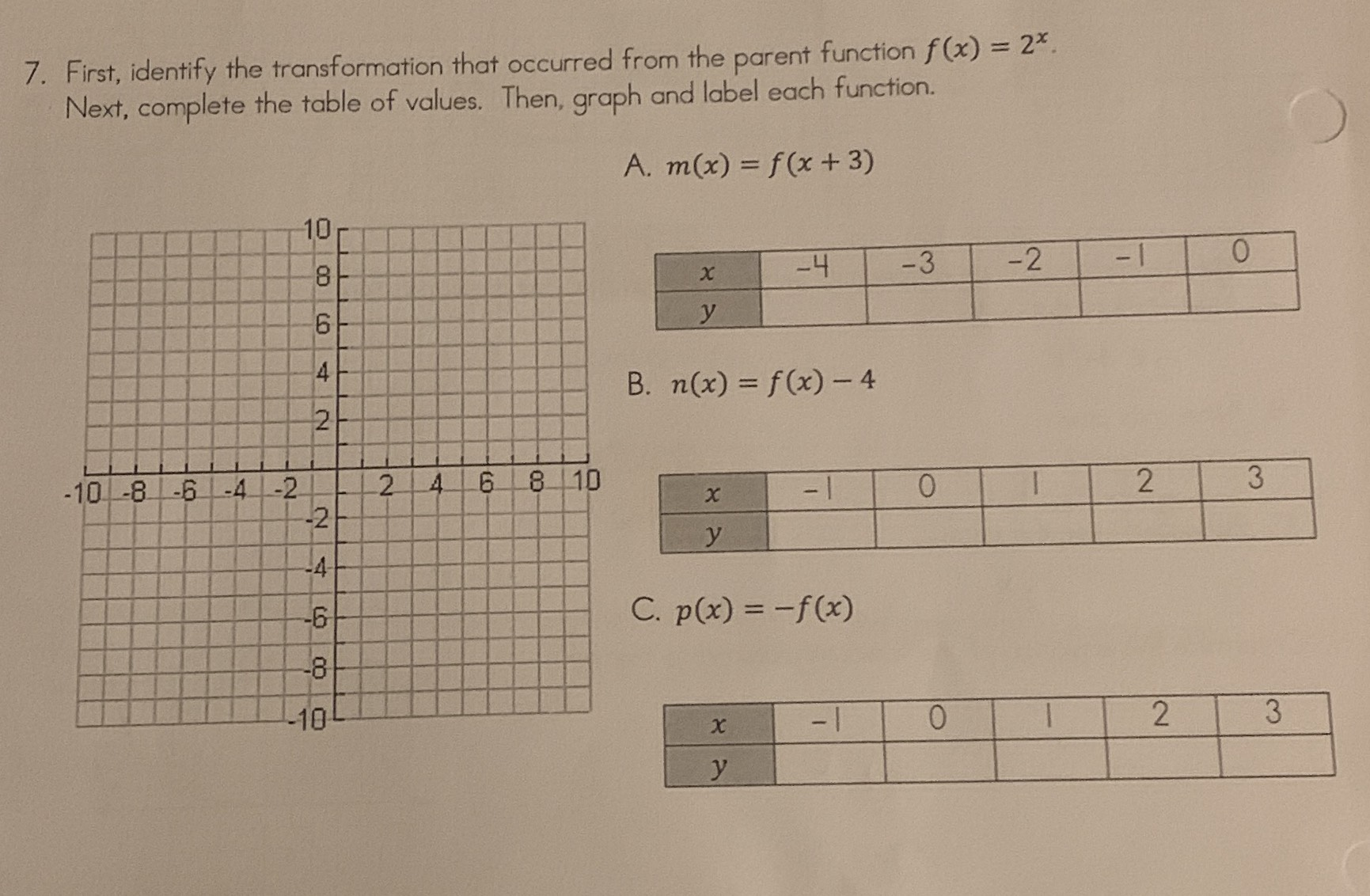### ¿Todavía tienes preguntas de matemáticas?

Pregunte a nuestros tutores expertos
Algebra
Pregunta7. First, identify the transformation that occurred from the parent function $$f ( x ) = 2 ^ { x }$$ . Next, complete the table of values. Then, graph and label each function.

A. $$m ( x ) = f ( x + 3 )$$

B. $$n ( x ) = f ( x ) - 4$$

C. $$p ( x ) = - f ( x )$$

A -4    -3     -2      -1     0

0.5   1     2         4        8

B    -1    0   1    2    3

-3.5    -3    -2   0   4

C  -1  0   1   2   3

-0.5  -1  -2  -4  -8

Solución
View full explanation on CameraMath App.